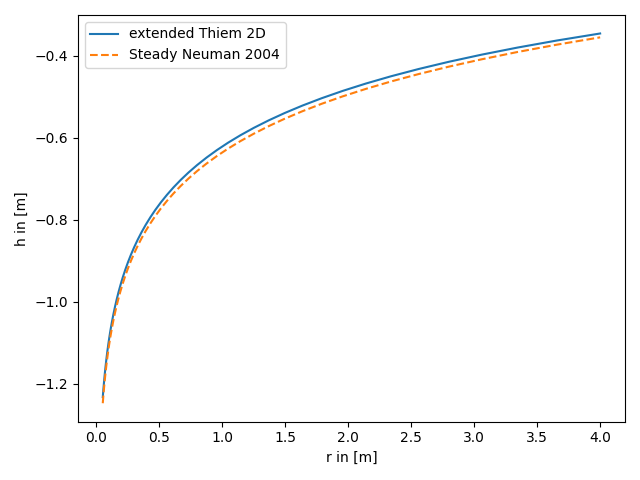# extended Thiem 2D vs. steady solution for apparent transmissivity from Neuman

Both, the extended Thiem and the Neuman solution, represent an effective steady drawdown in a heterogeneous aquifer. In both cases the heterogeneity is represented by two point statistics, characterized by mean, variance and length scale of the log transmissivity field. Therefore these approaches should lead to similar results.

References:```import numpy as np
from matplotlib import pyplot as plt

rad = np.geomspace(0.05, 4)  # radius from the pumping well in [0, 4]
r_ref = 30.0  # reference radius

var = 0.5  # variance of the log-transmissivity
len_scale = 10.0  # correlation length of the log-transmissivity
TG = 1e-4  # the geometric mean of the transmissivity

rate = -1e-4  # pumping rate